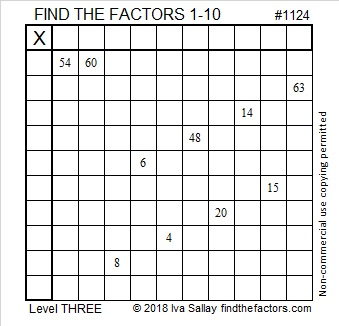# 1124 and Level 3

The common factors of 54 and 60 are 1, 2, 3, and 6. Just one of those common factors will put only numbers from 1 to 10 in the top row. That’s the factor you need to choose. To complete the puzzle, all the numbers from 1 to 10 must go in both the first column and the top row. Can you solve this puzzle?Print the puzzles or type the solution in this excel file: 10-factors-1121-1133

Here are a few facts about the number 1124:

• 1124 is a composite number.
• Prime factorization: 1124 = 2 × 2 × 281, which can be written 1124 = 2² × 281
• The exponents in the prime factorization are 2 and 1. Adding one to each and multiplying we get (2 + 1)(1 + 1) = 3 × 2  = 6. Therefore 1124 has exactly 6 factors.
• Factors of 1124: 1, 2, 4, 281, 562, 1124
• Factor pairs: 1124 = 1 × 1124, 2 × 562, or 4 × 281
• Taking the factor pair with the largest square number factor, we get √1124 = (√4)(√281) = 2√281 ≈ 33.526111124 is the hypotenuse of a Pythagorean triple:
640-924-1124 which is 4 times 160-231-281

If I asked you to tell me what is significant about this set of numbers {13, 16, 19, 22}, what would you say?

Perhaps you would tell me they make an arithmetic sequence in which the common difference is 3.

What you probably wouldn’t tell me is that 1124 is a palindrome in those four bases!
It’s 686 in BASE 13 because 6(13²) + 8(13) + 6(1) = 1124,
464 in BASE 16 because 4(16²) + 6(16) + 4(1) = 1124
323 in BASE 19 because 3(19²) + 2(19) + 3(1) = 1124, and
272 in BASE 22 because 2(22²) + 7(22) + 2(1) = 1124